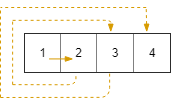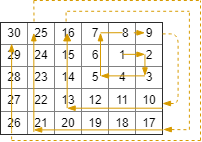# 螺旋矩阵 III

## 885. 螺旋矩阵 III (Medium)

```输入：R = 1, C = 4, r0 = 0, c0 = 0```

```输入：R = 5, C = 6, r0 = 1, c0 = 4```

1. `1 <= R <= 100`
2. `1 <= C <= 100`
3. `0 <= r0 < R`
4. `0 <= c0 < C`

[数组] [矩阵] [模拟]